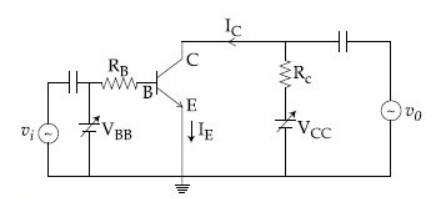Q

# Please help! - In the given figure, given that VBBsupply can vary from 0 to 5.0 V,, The minimum base current an - Electronic devices - JEE Main

In the given figure, given that VBB supply can vary from 0 to 5.0 V,  , The minimum base current and the input voltage at which the transistor will go to saturation, will be, respectively :• Option 1)

25  and 3.5 V

• Option 2)

25  and 2.8 V

• Option 3)

20  and 2.8 V

• Option 4)

20  and 3.5 V

Views

Relation between emitter current ,Base current,collector current -

- wherein

At saturation

We known that

So

So

Option 1)

25  and 3.5 V

Option 2)

25  and 2.8 V

Option 3)

20  and 2.8 V

Option 4)

20  and 3.5 V

Exams
Articles
Questions